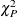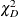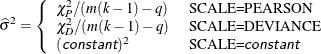Rescaling the Covariance Matrix

One way of correcting overdispersion is to multiply the covariance matrix by a dispersion parameter. You can supply the value of the dispersion parameter directly, or you can estimate the dispersion parameter based on either the Pearson’s chi-square statistic or the deviance for the fitted model.

The Pearson’s chi-square statisticand the devianceare defined in the section Lack-of-Fit Tests. If the SCALE= option is specified in the MODEL statement, the dispersion parameter is estimated byIn order for the Pearson’s statistic and the deviance to be distributed as chi-square, there must be sufficient replication within the subpopulations. When this is not true, the data are sparse, and the p-values for these statistics are not valid and should be ignored. Similarly, these statistics, divided by their degrees of freedom, cannot serve as indicators of overdispersion. A large difference between the Pearson’s statistic and the deviance provides some evidence that the data are too sparse to use either statistic.

You can use the AGGREGATE (or AGGREGATE=) option to define the subpopulation profiles. If you do not specify this option, each observation is regarded as coming from a separate subpopulation. For events/trials syntax, each observation represents n Bernoulli trials, where n is the value of the trials variable; for single-trial syntax, each observation represents a single trial. Without the AGGREGATE (or AGGREGATE=) option, the Pearson’s chi-square statistic and the deviance are calculated only for events/trials syntax.

Note that the parameter estimates are not changed by this method. However, their standard errors are adjusted for overdispersion, affecting their significance tests.